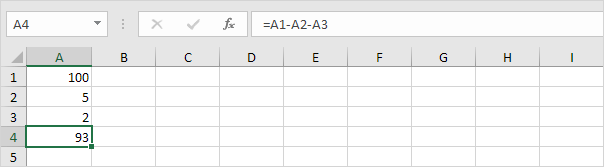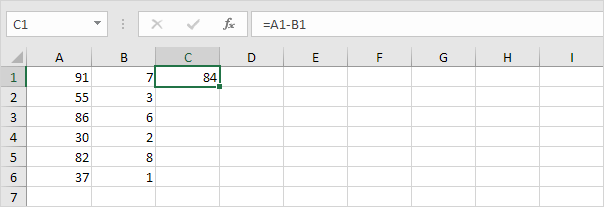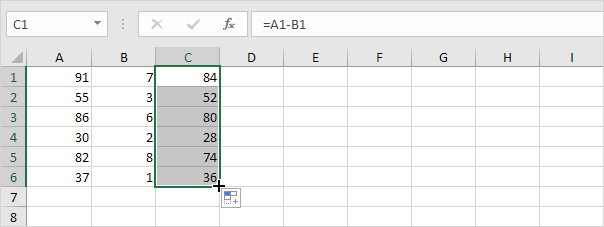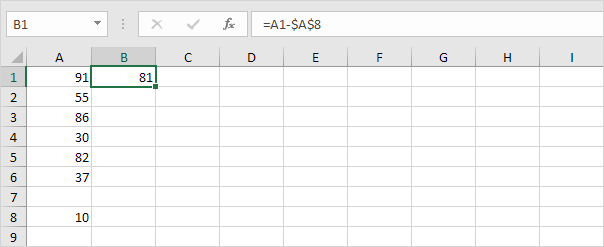## Subtraction

### Subtraction

Looking for SUBTRACT function in Excel?. There is no such function however, there are several ways to subtract numbers in Excel. If Are you ready to improve your Excel skills?, then this post is for you.

Quick Explanation: For subtraction select the cell(rows/columns) – other cell
(rows/columns). Here you can select single cell or multiple cells for subtraction.

1. Here is a simple example, enter numbers you want to subtract in a cell by Simply using the minus sign (-). Don’t forget to start a formula always with an equal sign (=).Simple and direct Subtraction

2. Here the number in cell A1 subtracts the value in cell A2 and the value in cell A3. At A4 we need to enter the subtraction formula to get the answer.Subtraction of Rows

3. As you can see, there are many cells are there to subtract so simply use the SUM function to shorten your formula. For instance, the formula below subtracts the values of cells in the range A2:A9 from the value in cell A1.Subtraction from a sum of amount

Checkout the screenshot below. To subtract the numbers of column B with the numbers of column A, we execute the following steps.

4a. First select cell C1, then subtract the value of cell A1 with the value of cell B1.Subtraction of Two Columns single cell

4b. To generate subtraction for all cells simply click on the lower right corner of cell C1 and drag it down to cell C6.Subtraction of two columns multiple cells

Take a look at the screenshot below. To subtract a number from a range of cells, execute the following steps.

5a. We have values to be subtracted in column A, so on column B1 Subtract the value of cell A1 with the value of cell A8. Put the reference to cell A8 by placing a \$ symbol in front of the column letter and row number (\$A\$8).Subtraction of same Rows in other column

5b. To get Subtraction for remaining cell, click on the lower right corner of cell B1 and drag it down to cell B6.